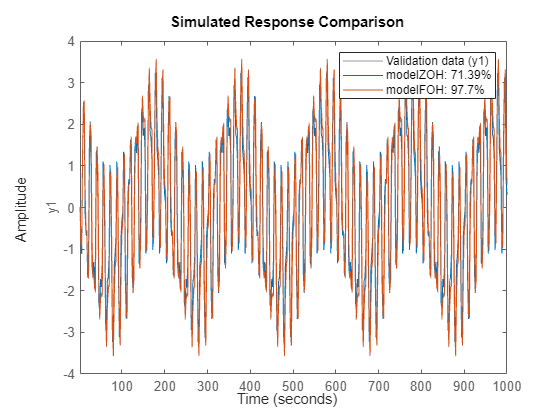## Effect of Input Intersample Behavior on Continuous-Time Models

The intersample behavior of the input signals influences the estimation, simulation and prediction of continuous-time models. A sampled signal is characterized only by its values at the sampling instants. However, when you apply a continuous-time input to a continuous-time system, the output values at the sampling instants depend on the inputs at the sampling instants and on the inputs between these points.

The `iddata` and `idfrd` objects have an `InterSample` property which stores how the input behaves between the sampling instants. You can specify the behavior between the samples to be piecewise constant (zero-order hold), linearly interpolated between the samples (first-order hold) or band-limited. A band-limited intersample behavior of the input signal means:

• A filtered input signal (an input of finite bandwidth) was used to excite the system dynamics.

• The input was measured using a sampling device (A/D converter with antialiasing) that reported it to be band-limited even though the true input entering the system was piecewise constant or linear. In this case, the sampling devices can be assumed to be a part of the system being modeled.

When the input signal is a band-limited discrete-time frequency-domain data (`iddata` with `domain = 'frequency'` or `idfrd` with sample time Ts≠0), the model estimation is performed by treating the data as continuous-time data (Ts = 0). For more information, see Pintelon, R. and J. Schoukens, System Identification. A Frequency Domain Approach, section 10.2, pp-352-356,Wiley-IEEE Press, New York, 2001.

The intersample behavior of the input data also affects the results of simulation and prediction of continuous-time models. `sim` and `predict` commands use the `InterSample` property to choose the right algorithm for computing model response.

The following example simulates a system using first-order hold ( `foh` ) intersample behavior for input signal.

```sys = idtf([-1 -2],[1 2 1 0.5]); rng('default') u = idinput([100 1 5],'sine',[],[],[5 10 1]); Ts = 2; y = lsim(sys,u,(0:Ts:999)','foh');```

Create an `iddata` object for the simulated input-output data.

`data = iddata(y,u,Ts);`

The default intersample behavior is zero-order hold ( `zoh` ).

`data.InterSample`
```ans = 'zoh' ```

Estimate a transfer function using this data.

```np = 3; % number of poles nz = 1; % number of zeros opt = tfestOptions('InitializeMethod','all','Display','on'); opt.SearchOptions.MaxIterations = 100; modelZOH = tfest(data,np,nz,opt)```
```modelZOH = From input "u1" to output "y1": -217.2 s - 391.6 --------------------------------- s^3 + 354.4 s^2 + 140.2 s + 112.4 Continuous-time identified transfer function. Parameterization: Number of poles: 3 Number of zeros: 1 Number of free coefficients: 5 Use "tfdata", "getpvec", "getcov" for parameters and their uncertainties. Status: Estimated using TFEST on time domain data "data". Fit to estimation data: 81.38% FPE: 0.1146, MSE: 0.111 ```

The model gives about 80% fit to data. The sample time of the data is large enough that intersample inaccuracy (using `zoh` rather than `foh` ) leads to significant modeling errors.

Re-estimate the model using `foh` intersample behavior.

```data.InterSample = 'foh'; modelFOH = tfest(data,np,nz,opt)```
```modelFOH = From input "u1" to output "y1": -1.197 s - 0.06843 ------------------------------------- s^3 + 0.4824 s^2 + 0.3258 s + 0.01723 Continuous-time identified transfer function. Parameterization: Number of poles: 3 Number of zeros: 1 Number of free coefficients: 5 Use "tfdata", "getpvec", "getcov" for parameters and their uncertainties. Status: Estimated using TFEST on time domain data "data". Fit to estimation data: 97.7% FPE: 0.001748, MSE: 0.001693 ```

`modelFOH` is able to retrieve the original system correctly.

Compare the model outputs with data.

`compare(data,modelZOH,modelFOH)``modelZOH` is compared to data whose intersample behavior is `foh`. Therefore, its fit decreases to around 70%.